Courses

# RS Aggarwal Solutions: Whole Numbers Exercise - 3C Notes | EduRev

## Class 6 : RS Aggarwal Solutions: Whole Numbers Exercise - 3C Notes | EduRev

The document RS Aggarwal Solutions: Whole Numbers Exercise - 3C Notes | EduRev is a part of the Class 6 Course Mathematics (Maths) Class 6.
All you need of Class 6 at this link: Class 6

Q.1. Perform the following subtractions. Check your results by the
corresponding additions :
(i) 6237 – 694
(ii) 21205 – 10899
(iii) 100000 – 78987
(iv) 1010101 – 656565
Ans.
(i)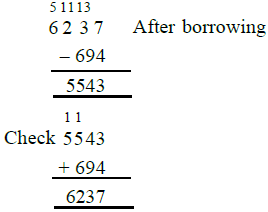(ii)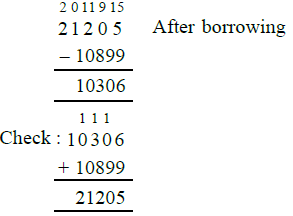(iii)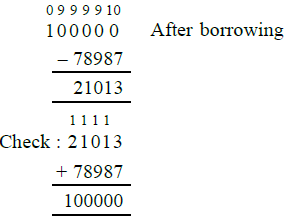(iv)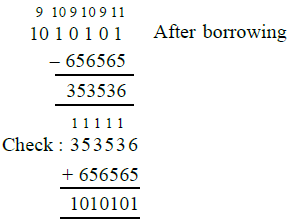Q.2. Replace each * by the correct digit in each of the following :
(i)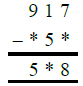(ii)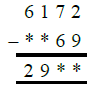(iii)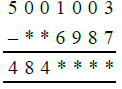(iv)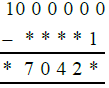Ans.
(i)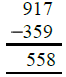(ii)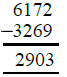(iii)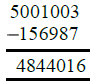(iv)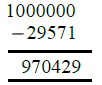Q.3. Find the difference :
(i) 463 – 9
(ii) 5632 – 99
(iii) 8640 – 999
(iv) 13006 – 9999
Ans. (i) 463 – 9 = 464 – 1 – 9 = 464 – 10
= 454
(ii) 5632 – 99 = 5632 – 100 + 1
= 5633 – 100 = 5533
(iii) 8640 – 999 = 8640 – 1000 + 1
= 8641 – 1000 = 7641
(iv) 13006 – 9999 = 13006 – 10000 + 1
= 13007 – 10000 = 12007

Q.4. Find the difference between the smallest number of 7 digits and the largest number of 4 digits.
Ans. Smallest number of 7-digits = 1000000
Largest number of 4-digits = 9999
Required difference = (1000000 – 9999)
= 990001

Q.5. Ravi opened his account in a bank by depositing Rs. 136000. Next day he

withdrew Rs. 73129 from it. How much money was let in his account ?
Ans. Deposit in the beginning = Rs. 136000
Next day withdrew = Rs. 73129
∴ Amount left in the bank account
= Rs. 136000 – 73129 = Rs. 62871

Q.6. Mrs Saxena withdrew Rs. 100000 from her bank account. She purchased a TV

set for Rs. 38750, a refrigerator for Rs. 23890 and jewellery worth Rs. 35560. How much money was left with her ?
Ans. Amount withdrawn from the bank
= Rs. 1,00,000
Cost of TV set = Rs. 38750
Cost of refrigerator = Rs. 23890
Cost of jewellery = Rs. 35560
Total amount spent on her purchase
= Rs. (38750 + 23890 + 35560)
= Rs. 98200
Amount left with her = Rs. 1,00,000 –
Rs. 98200 = Rs. 1800

Q.7. The population of a town was 110500. In one year it increased by 3608 due to new births. However 8973 persons died or left the town during the year. What was the population at the end of the year?
Ans. Population of a town = 110500
Increase in 1 year = 3608
Persons left or died = 8973
∴ The population at the end of year
= 110500 + 3608 – 8973
= 114108 – 8973 = 105135

Q.8. Find the whole number n when :
(i) n + 4 = 9

(ii) n + 35 = 101
(iii) n – 18 = 39
(iv) n – 20568 = 21403
Ans. (i) We have n + 4 = 9
n = 9 – 4 = 5
∴ n = 5
(ii) n + 35 = 101
Subtracting 35 from both sides
n + 35 – 35 = 101 – 35 ⇒ n = 66
∴ n = 66
(iii) n – 18 = 39
Adding 18 to both sides
n – 18 + 18 = 39 + 18
⇒ n = 57
∴ n = 57

(iv) n – 20568 = 21403
Adding 20568 to both sides
n – 20568 + 20568 = 21403 + 20568
n = 41971

Offer running on EduRev: Apply code STAYHOME200 to get INR 200 off on our premium plan EduRev Infinity!

## Mathematics (Maths) Class 6

191 videos|220 docs|43 tests

,

,

,

,

,

,

,

,

,

,

,

,

,

,

,

,

,

,

,

,

,

;